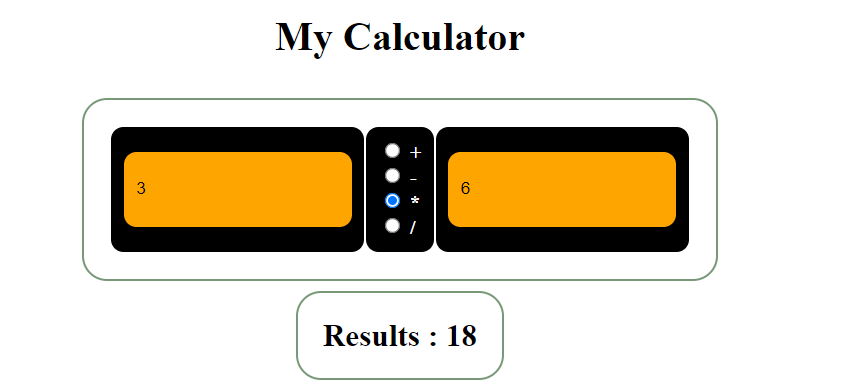### Simple Online Calculator Website

Question
Implement a simple online calculator, using HTML, CSS, and JavaScript.

Requirements:

1. The calculator should support addition, substraction,multiplication,and division.
2. The result should be automatically computed whenever either operand is changed.
3. The Result should be automatically computed whenever the operator is changed
4. The division by zero should be handled appropriately.
5. It should be in ure JavaScript, HTML, (and CSS). No External Library would be allowed
6. Layout and Code format

Solution:
Code <html><head> <title>My Calculator</title> <link rel="stylesheet" type="text/css" href="calculatorDemo.css" /> </head> <body> <h1>My Calculator</h1> <table> <tr> <td><input id="num1" type="text" name="num1" placeholder="Number 1"></td> <td style="color:#ffffff; font-weight:700;"> <input id="add" name="operate" type="radio" value="+" /> + <br> <input id="sub" name="operate" type="radio" value="-" /> - <br> <input id="multi" name="operate" type="radio" value="*" /> * <br> <input id="divide" name="operate" type="radio" value="/" /> / </td> <td><input id="num2" type="text" name="num2" placeholder="Number 2"></td> </tr>